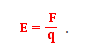# Unit of Electric Field

Before getting into the unit of electric field it is very important to have a good knowledge about what an electric field is. Let’s start with the definition. An electric field is a field or space around an electrically charged object where other electrical objects experience a certain force. Additionally, an electric field can also be defined as an electric property that is associated with a certain point in space when some form of charge is present.

Moving on, an electric field is a vector quantity meaning it has magnitude and direction. It is further expressed by the value of E, which is known as electric field strength or electric field intensity. It is represented by the equation;Where f = force acting in newtons and q = charge in coulombs.

Let’s look at the units below.

## SI Unit of Electric Field

The SI unit of the electric field is volts per meter (V/m). This unit is equivalent to Newton’s per coulomb. These are derived units where Newton is a unit of force and Coulomb is the unit of charge.